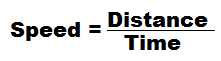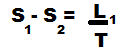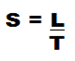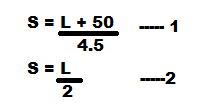# Problems based on Trains - Revision with ExamplesProblems based on train is a part of Time and Distance chapter but moving and stationary objects make this chapter somewhat different.  Today I am going to help you revise this chapter using simple examples.

### Formula:#### Some useful concepts :-

I. To change k/h in m/s we multiply by 5/18

E.g. 72 k/h means
72 × 5/18 = 4 × 5 = 20 m/s

II. To change m/sec in k/h we multiply by 18/5

E.g. 45m/s means
45 × 18/5 = (9 × 18) k/h = 162 k/h

III. In the concept of trains, there are two objects. First object train and second is that which is crossed by the train.

#### Tips:

Case 1 – When a moving train crosses a man, the first object is train and second object is man.

Case 2 – When a moving train crosses a platform, first object is train and second is platform.

Case 3 – When a moving train crosses a man who is standing on a railway platform, first object is train and second is man.

Case 4 – When a moving train crosses another moving train (same or opposite) direction; the first object i s first train and second is second train.

Case 5 – When a moving train crosses a man who is standing in another moving train, the first object is first train and second one is man (not 2nd train)

Rule I: When a train crosses a man/pole/tree (in stationary form)

Let a train is having a speed is S and the length L, crosses a pole in T time then,Here the breadth of man, tree, pole is negligible with respect to the train.

Rule II: When train crosses a platform.

Let a train having speed of S and length L, crosses a platform whose length is L2, in time T, thenRule III : When a train crosses another running train

Let a train having Speed S, and length L, crosses another train which is travelling in opposite direction at a speed of S2 and L2 in time T. Then-Both object having length and speed.

NOTE:

(i) If both object having length (L1 and L2) then, the Length is always sum i.e. (L1 and L2)

(ii) If both object having speed S1 and S2, then the speed are added. (When direction is opposite) and subtracted (When direction is same)

Rule IV:  When a moving train crosses a man who is running

Let a train having a speed S1 and length L, crosses a man, who is running at a speed S2 in the same direction T time, thenRule V: When a moving train crosses a man who is sitting into a moving train.

Let a train having a speed S1 and length L1 crosses a man who is sitting in another train which is travelling in opposite directions at a speed of S2 and L2 in time T### EXAMPLES:

1) Find the time taken by a train 180m long, running at 72 km/h in crossing an electrical pole.

Solution –2) A train 140m is running at 60 km/h. In how much time will it pass a platform 260m long?

Solution:3) A train 100m long is running at a speed of 70 km/h. In what time will it pass a man who is running at a speed of 10 km/h in the same direction?

Solution:4) A train 160m long taken 30 sec in crossing a tunnel 440m long. The speed of the train is

Solution:5) A man is standing on a railway bridge which is 50m long. He finds that a train is crossed on the bridge in 4 ½ sec but himself in 2 sec. Find the length and speed of train

Solution:From 1 and 24.5 L = 2 L + 100
2.5 L = 100 = L =40m
S= 40/2
= 20m/s

==>> Must read - Time and Distance concept